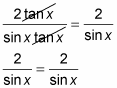##### Trigonometry For Dummies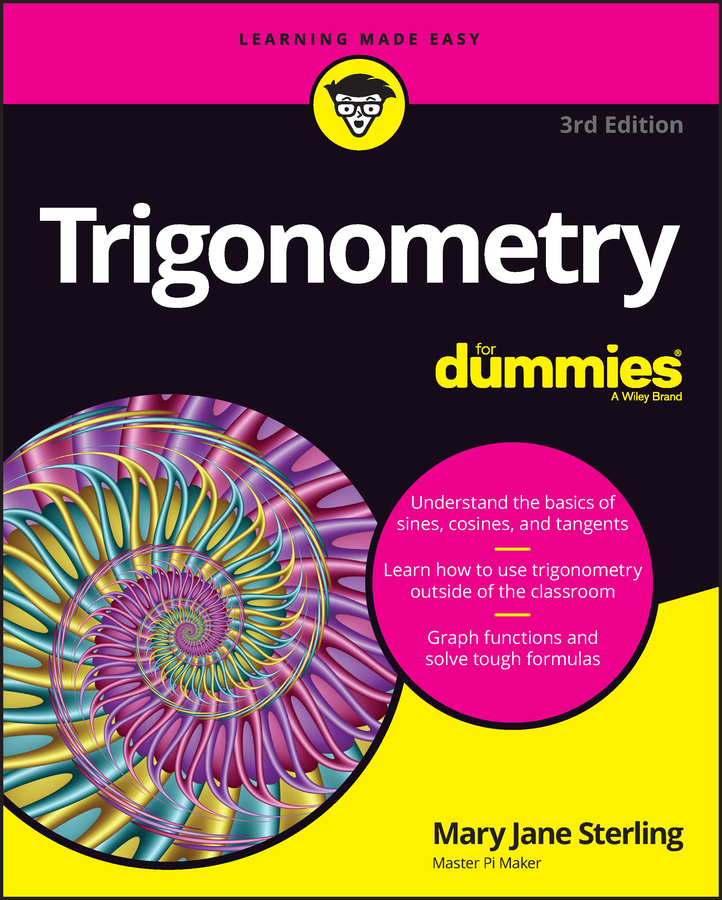A trig identity with fractions can work to your advantage; you’re given a plan of attack. You can work toward getting rid of the fraction and, in the process, solve the problem. Two of the main techniques for working with fractions in identities are: (1) break them up into separate terms, or (2) to go in the other direction and find a common denominator.

Breaking up fractions isn’t hard to do. In fact, when you can, doing so is one of the easiest and most productive ways to solve identities. The trick is to break them up correctly. You can break up a fraction that has several terms in the numerator and one in the denominator — but not the other way around.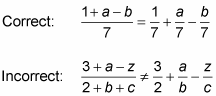Now apply this breaking up of fractions to a trigonometry identity. In this first example, the fraction on the left has just one term in the denominator. Solve the identity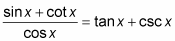1. Break up the fraction by writing each term over the denominator.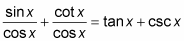2. Rewrite cot x by using the ratio identity.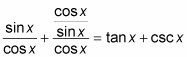3. Simplify the complex fraction by flipping the denominator and multiplying it by the numerator.

Then reduce the result.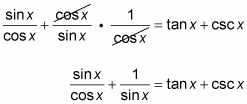4. Replace the first fraction by using the ratio identity for tangent and the second fraction by using the reciprocal identity for cosecant.

tan x + csc x = tan x + csc x

You can also break up fractions that have more than one factor (but only one term) in the denominator by carrying them both along. For example, solve the identity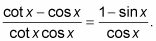1. Break up the fraction by writing each term in the numerator over the entire denominator.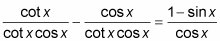2. Reduce each fraction on the left side.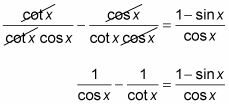3. Rewrite cot x in the second denominator by using the ratio identity.

Then simplify the complex fraction by flipping the denominator and multiplying.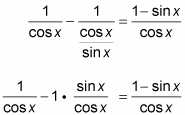The two fractions now have the same denominator.

4. Rewrite the left side as all one fraction.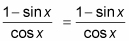Combining fractions by finding a common denominator often produces very nice results. For example, solve the identity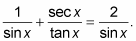1. Find a common denominator for the fractions on the left and rewrite the fractions.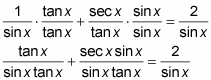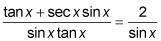3. Replace sec x using its reciprocal identity.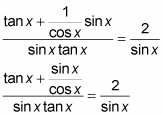4. Use the ratio identity to replace the second term in the numerator — then simplify.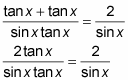5. Reduce the fraction.